Get instant live expert help with Excel or Google Sheets“My Excelchat expert helped me in less than 20 minutes, saving me what would have been 5 hours of work!”

#### Post your problem and you’ll get Expert help in seconds.

Your message must be at least 40 characters
Our professional Expert are available now. Your privacy is guaranteed.

# How to convert dates to month and year

We can use the TEXT function to make Excel convert dates to month and years.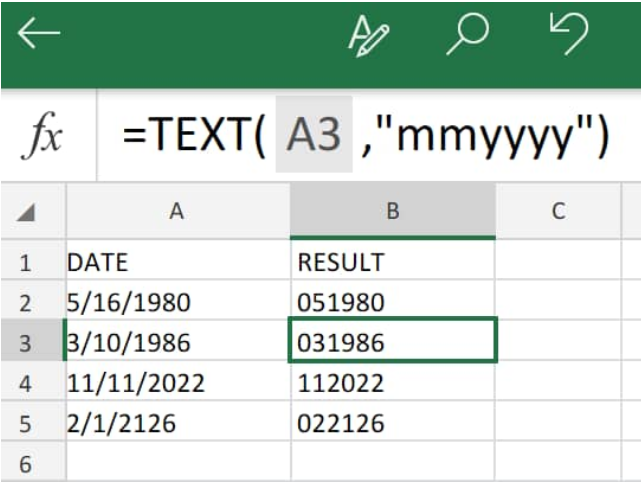Figure 1. Text Function in Excel

## Generic Formula

`=TEXT(date,"mmyyyy")`

If we want to convert an Excel date to month and year  format (e.g. 05/19/2019 > 052017), we can use TEXT function.

## How to Change Dates to Month and Year in Excel

We will have to generate an Excel formula to convert dates to month and year

Our formula will be based on the TEXT Function.

The TEXT function in Excel applies our specified number format to a number value, and generates a text result.

Here’s how to make Excel convert to month and year only;

1. We begin by gathering the number values we want to convert into our spreadsheet;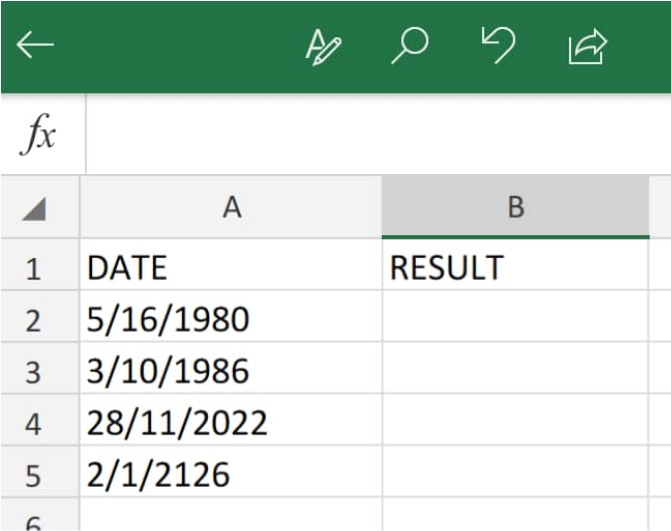Figure 2. Dates in Excel

Be sure to provide an empty column of cells for Excel to return the converted results (Column B above)

1. Our formula for Excel to convert date to month and year is as follows;

`=TEXT(A2,”MMYYYY”)`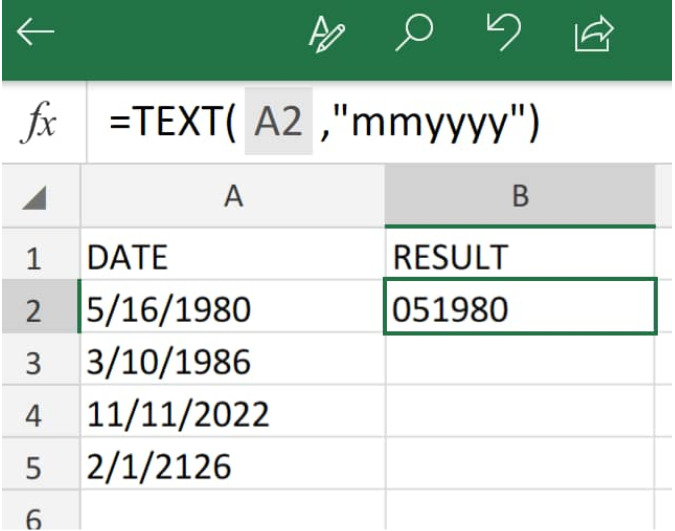Figure 3. Convert Dates in Excel

1. Modify and copy the Excel formula to convert dates to month and year (in cell B2 above) down into the other columns in column B to get similar results;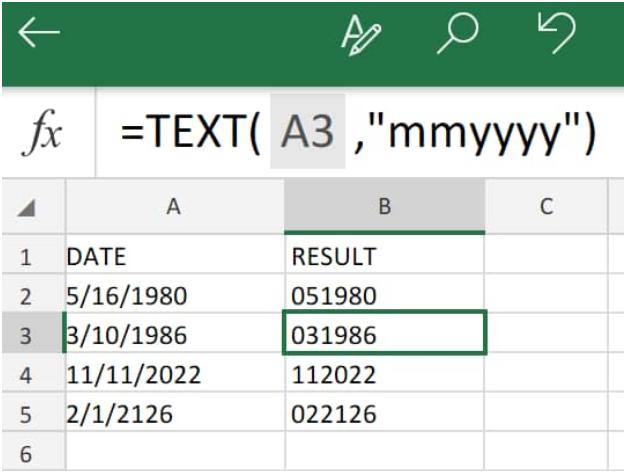Figure 4. Convert Dates in Excel

## How to Convert a Date to a Month in Excel

If our goal is to convert dates to months only, we will simply adjust the components of our TEXT formula to convert dates to month and year (used above).

Our Excel formula to convert date to month in cell B5 below is as follows;

`=TEXT(A5,mm)`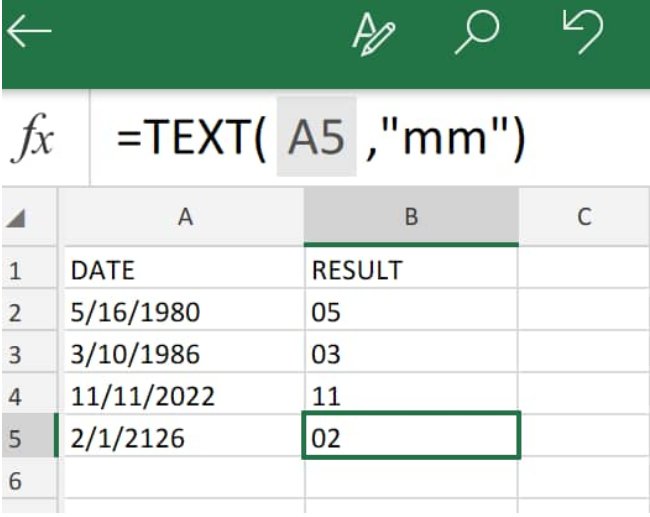Figure 5. Convert Dates to Month in Excel

## How to Convert Date to Year in Excel

If we want to make Excel convert dates to year only, we modify our TEXT formula as follows;

`=TEXT(A2,”yyyy”)`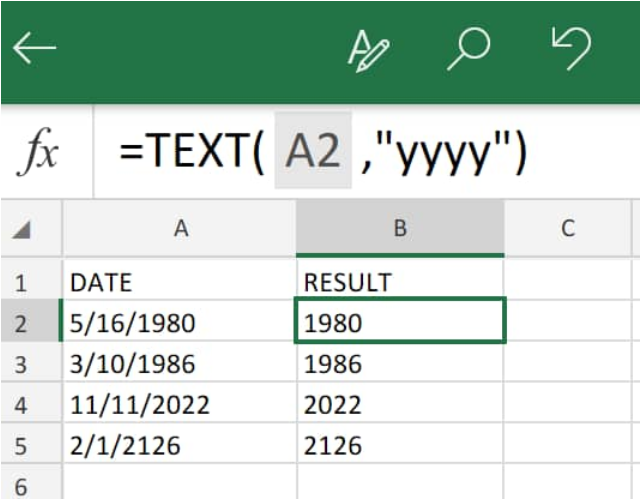Figure 6. Convert Dates to Year in Excel

## Instant Connection to an Excel Expert

Most of the time, the problem you will need to solve will be more complex than a simple application of a formula or function. If you want to save hours of research and frustration, try our live Excelchat service! Our Excel Experts are available 24/7 to answer any Excel question you may have. We guarantee a connection within 30 seconds and a customized solution within 20 minutes.